# Lineplot using Seaborn in Python

• Last Updated : 21 Oct, 2021

Seaborn is an amazing visualization library for statistical graphics plotting in Python. It provides default styles and color palettes to make statistical plots more attractive. It is built on the top of the matplotlib library and is also closely integrated into the data structures from pandas.

## Lineplot

Visual representation of a dataset must be chosen according to the dataset or the type of answer we want from the plot. Scatter plots are highly preferred for visualizing statistical relationships. But when it comes to data which is varying with time (or continuous variable), scatter plots are not a good choice. Instead, in Seaborn, lineplot() or relplot() with kind = ‘line’ must be preferred. Line plots give annotation to each of the points and plus helps in customizing markers, line style, and legends.

Syntax: seaborn.lineplot(x=None, y=None, hue=None, size=None, style=None, data=None, palette=None, hue_order=None, hue_norm=None, sizes=None, size_order=None, size_norm=None, dashes=True, markers=None, style_order=None, units=None, estimator=’mean’, ci=95, n_boot=1000, seed=None, sort=True, err_style=’band’, err_kws=None, legend=’brief’, ax=None, **kwargs)
Parameters:
x, y: Input data variables; must be numeric.
data: Dataframe where each column is a variable and each row is an observation.
size: Grouping variable that will produce lines with different widths.
style: Grouping variable that will produce lines with different dashes and/or markers.

Example 1: Let’s take an example of FMRI dataset. It is an example of time-series data, where variables are a function of time. This dataset is in-built available and can be accessed using load_dataset() and needs not to be downloaded separately.

## Python3

 `# import libraries` `import` `seaborn as sns`   `# load dataset` `fmri ``=` `sns.load_dataset(``"fmri"``)`

There can be multiple measurements of the same variable. So we can plot the mean of all the values of x and 95% confidence interval around the mean. This behavior of aggregation is by default in seaborn.

## Python3

 `# plotting lineplot for signal with respect to timepoint` `# using relplot, kind = "line"` `# code added by devanshigupta1304` `sns.lineplot( x ``=` `"timepoint"``, ` `             ``y ``=` `"signal"``, ` `             ``data ``=` `fmri);`

Output-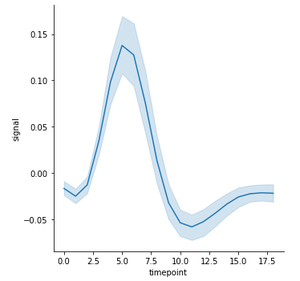Example 2: Creating multiple line plot using hue parameters.

## Python3

 `sns.lineplot( x ``=` `"timepoint"``, ` `             ``y ``=` `"signal"``, ` `             ``hue ``=` `"region"``,` `             ``data ``=` `fmri);`

Output: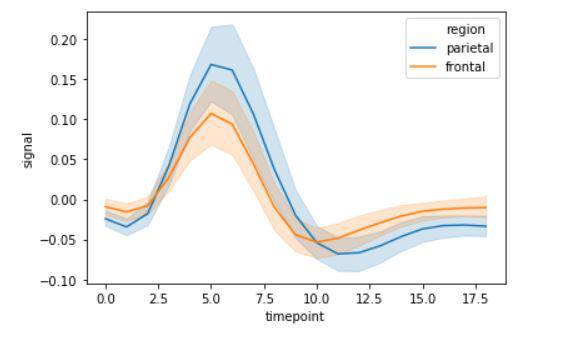Example 3: Creating multiple line plots using style parameters.

## Python3

 `sns.lineplot( x ``=` `"timepoint"``, ` `             ``y ``=` `"signal"``, ` `             ``hue ``=` `"region"``,` `             ``style ``=` `"event"``,` `             ``data ``=` `fmri);`

Output: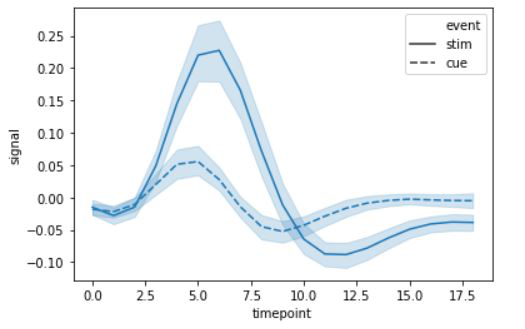Example 4: Creating multiple line plots using size parameters. We can use size attributes to change the line pattern.

## Python3

 `sns.lineplot( x ``=` `"timepoint"``, ` `             ``y ``=` `"signal"``, ` `             ``size ``=` `"event"``,` `             ``data ``=` `fmri);`

Output: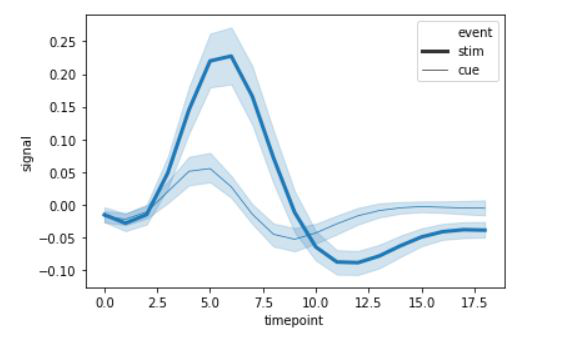Example 5: Grouping data points on the basis of category, here as region and event. We can add them as hue and style semantics. This will change the line pattern by default.

## Python3

 `# code added by devanshigupta1304` `# using relplot with kind = "line" ` `# adding style and hue semantic` `sns.relplot( x ``=` `"timepoint"``, ` `            ``y ``=` `"signal"``, ` `            ``hue ``=` `"region"``, style ``=` `"event"``,` `            ``kind ``=` `"line"``, data ``=` `fmri);`

Output: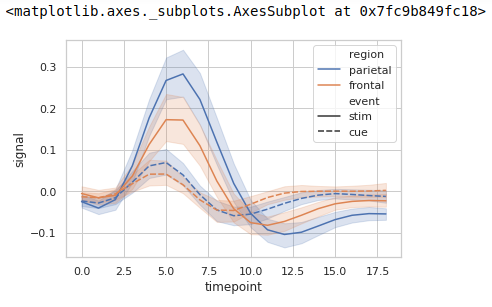Example 6: We can also represent standard deviation instead of confidence interval at each time point, for a large dataset, as it can be very time-consuming.

## Python3

 `# code added by devanshigupta1304` `# plotting lineplot for signal with respect to timepoint` `# using relplot, kind = "line"` `# using standard deviation instead of confidence interval` `sns.relplot(x ``=` `"timepoint"``, y ``=` `"signal"``, ` `            ``kind ``=` `"line"``, ci ``=` `"sd"``, ` `            ``data ``=` `fmri);`

Output –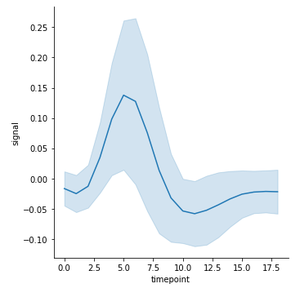Example 7: We can use style and hue with different columns in a data set.

## Python3

 `# using lineplot with style = column_name ` `# adding style and hue semantic` `sns.lineplot(x ``=` `"timepoint"``, y ``=` `"signal"``,` `             ``hue ``=` `"subject"``,style ``=` `"event"``,` `             ``data ``=` `fmri);`

Output: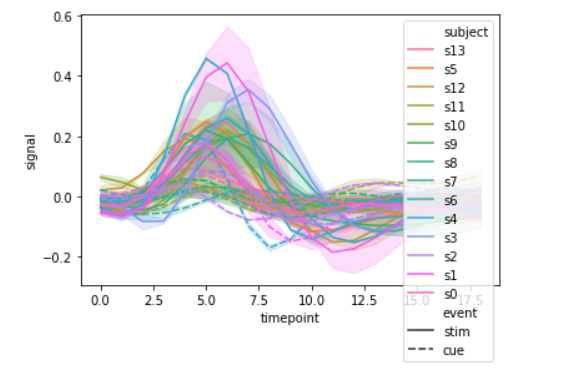Example 8: Using palette attributes

## Python3

 `# using lineplot ` `# adding palette, style and hue semantic` `sns.lineplot( x ``=` `"timepoint"``, y ``=` `"signal"``,` `             ``hue ``=` `"subject"``, style ``=` `"event"``,` `             ``palette ``=` `"YlOrRd_r"``, data ``=` `fmri);`

Output: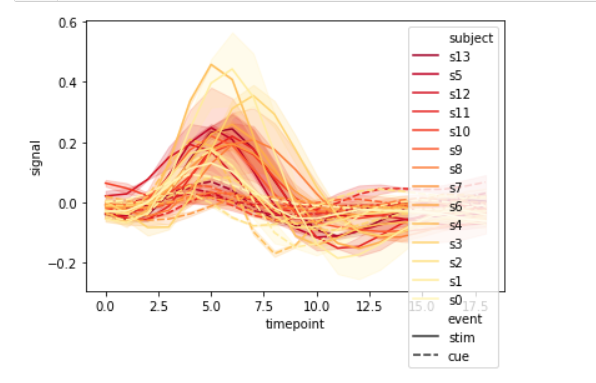Example 9: Adding error bar in line plot using err_style attributes.

## Python3

 `sns.lineplot( x ``=` `"timepoint"``, ` `             ``y ``=` `"signal"``, ` `             ``data ``=` `fmri,` `             ``err_style``=``"bars"``);`

Output: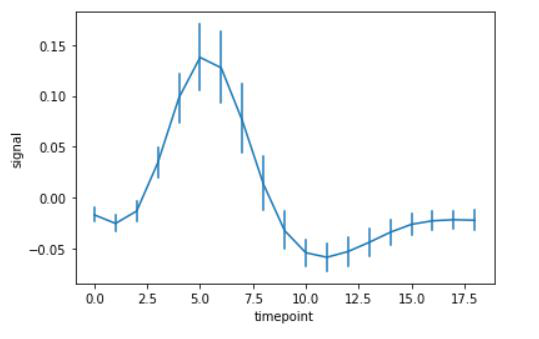My Personal Notes arrow_drop_up
Recommended Articles
Page :